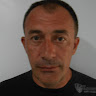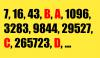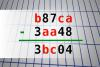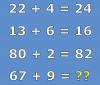BRAIN TEASERSBrain Teasers User Profile

# Miroslav Dimitrijevic

rank
197
points
26
See full ranking list
short ranking list
 195 Айдар Бусурманов 27 196 Islam Usman 26 197 Miroslav Dimitrijevic 26 198 Donald Wager 26 199 Nimish Mittal 25
 Calculate A+B-C+D Look at the series (7, 16, 43, B, A, 1096, 3283, 9844, 29527, C, 265723, D, ...), determine the pattern, and find the unknown values (A, B, C and D) and calculate A+B-C+D!Find number abc If b87ca - 3aa48 = 3bc04 find number abc. Multiple solutions may exist.Mathematical Puzzle: IF 22+4... Mathematical Puzzle: IF 22+4=24, 13+6=16 and 80+2=82 THEN 67+9=?